Ionic Compounds Writing Formulas Empirical Formulas formulas with

• Slides: 19Ionic Compounds: Writing FormulasEmpirical Formulas formulas with smallest whole-number ratio of elements in compound ionic compounds are only written as empirical formulas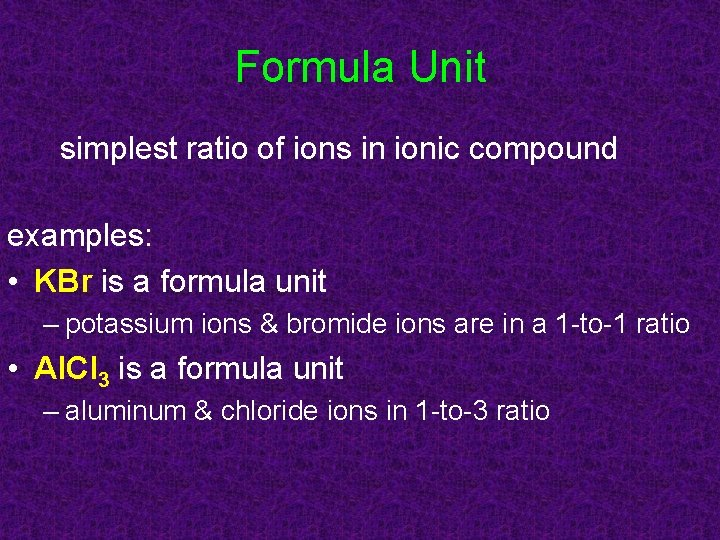Formula Unit simplest ratio of ions in ionic compound examples: • KBr is a formula unit – potassium ions & bromide ions are in a 1 -to-1 ratio • Al. Cl 3 is a formula unit – aluminum & chloride ions in 1 -to-3 ratio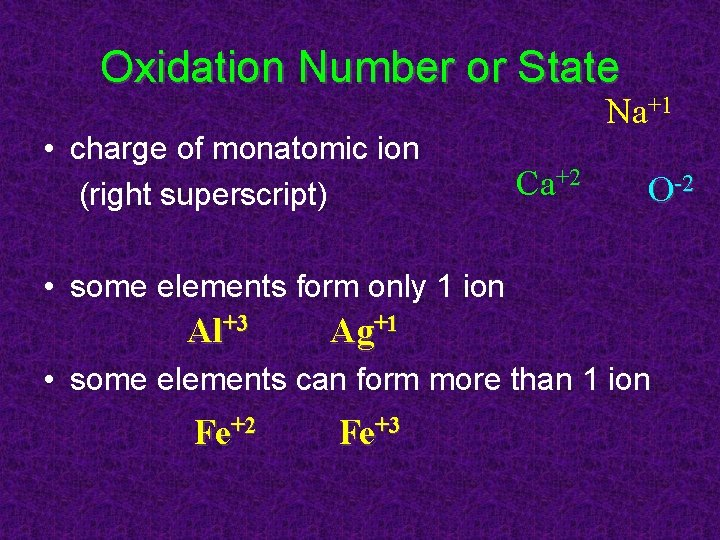Oxidation Number or State • charge of monatomic ion (right superscript) Na+1 Ca+2 O-2 • some elements form only 1 ion Al+3 Ag+1 • some elements can form more than 1 ion Fe+2 Fe+3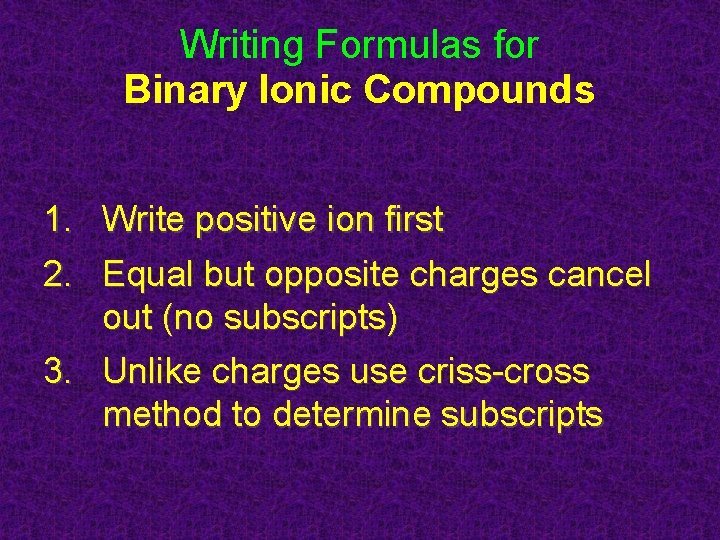Writing Formulas for Binary Ionic Compounds 1. Write positive ion first 2. Equal but opposite charges cancel out (no subscripts) 3. Unlike charges use criss-cross method to determine subscripts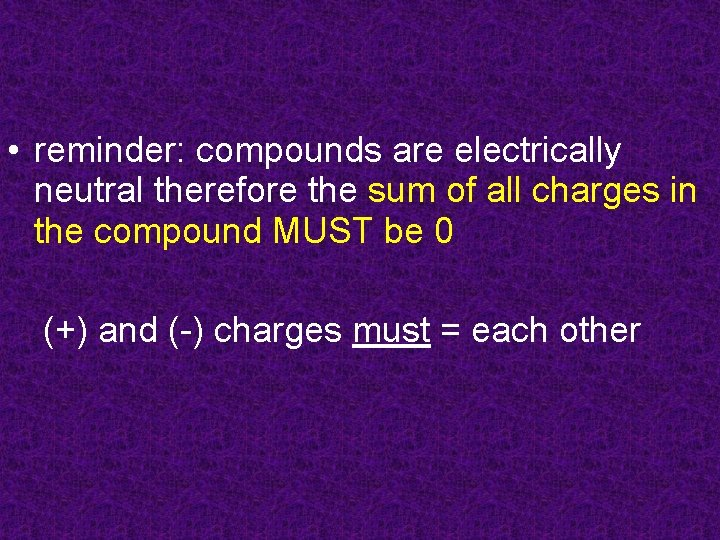• reminder: compounds are electrically neutral therefore the sum of all charges in the compound MUST be 0 (+) and (-) charges must = each otherEqual but Opposite Charges • Na+1 and Cl-1: Na. Cl +1 + (-1) = 0 • Mg+2 and O-2: Mg. O +2 + (-2) = 0 • Al+3 and P-3: +3 + (-3) = 0 Al. P • Formula: write symbols, (+) FIRST! FIRSTtry a few formulas: • • • Li+1 and I-1 Ca+2 and O-2 Al+3 and N-3 K+1 and F-1 Ba+2 and S-2 Li. I Ca. O Al. N KF Ba. SCriss-Cross Method charges opposite but NOT equal: • Mg+2 and Cl-1, CROSS and DROP! (# only, forget signs!) • Mg 1 Cl 2 (if subscript is 1, don’t write it!) Mg. Cl 2Check the Math • Mg. Cl 2 means 1 Mg+2 and 2 Cl-1 (1)(+2) + (2)(-1) = +2 + -2 = 0 • charges MUST add up to zero!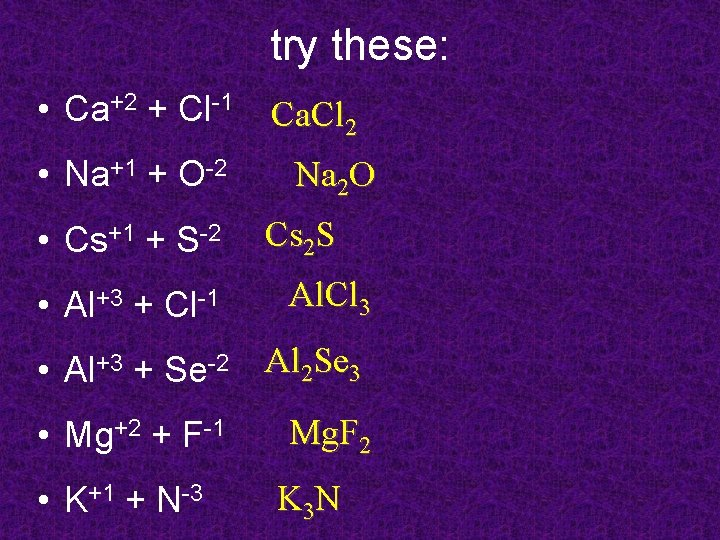try these: • Ca+2 + Cl-1 • Na+1 + O-2 • Cs+1 + S-2 • Al+3 + Cl-1 Ca. Cl 2 Na 2 O Cs 2 S Al. Cl 3 • Al+3 + Se-2 Al 2 Se 3 • Mg+2 + F-1 • K+1 + N-3 Mg. F 2 K 3 Nof course, it gets more difficult KF • potassium (K) and fluorine (F) • zinc (Zn) and iodine (I) Zn. I 2 Na 2 O • sodium (Na) and oxygen (O) Mg. O • magnesium (Mg) and oxygen (O) • aluminum (Al) and oxygen (O) Al 2 O 3 • calcium (Ca) and bromine (Br) Ca. Br 2 • cesium (Cs) and iodine (I) • silver (Ag) and sulfur (S) Cs. I Ag 2 S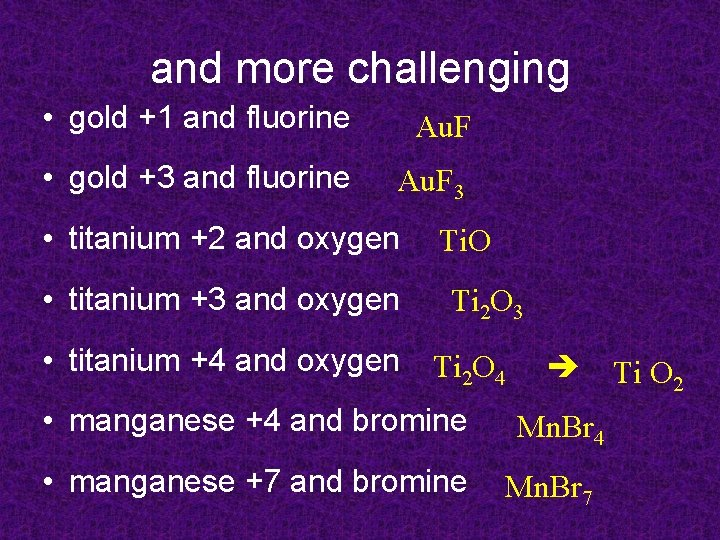and more challenging • gold +1 and fluorine Au. F • gold +3 and fluorine Au. F 3 • titanium +2 and oxygen • titanium +3 and oxygen • titanium +4 and oxygen Ti. O Ti 2 O 3 Ti 2 O 4 • manganese +4 and bromine • manganese +7 and bromine Ti O 2 Mn. Br 4 Mn. Br 7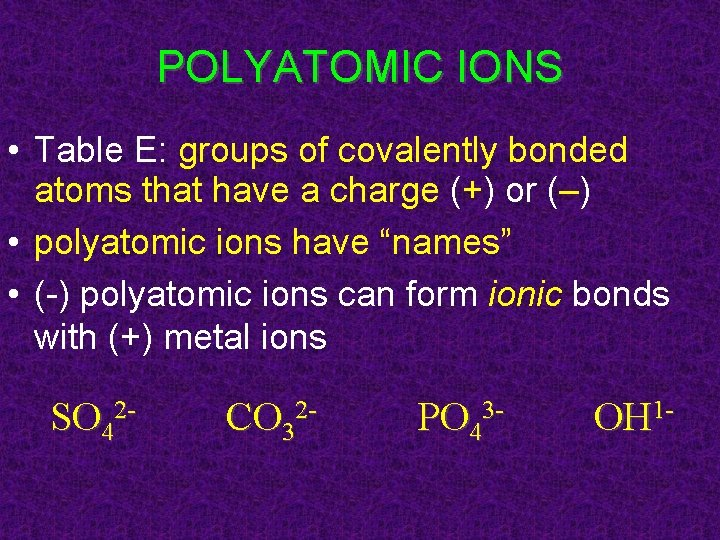POLYATOMIC IONS • Table E: groups of covalently bonded atoms that have a charge (+) or (–) • polyatomic ions have “names” • (-) polyatomic ions can form ionic bonds with (+) metal ions SO 42 - CO 32 - PO 43 - OH 1 -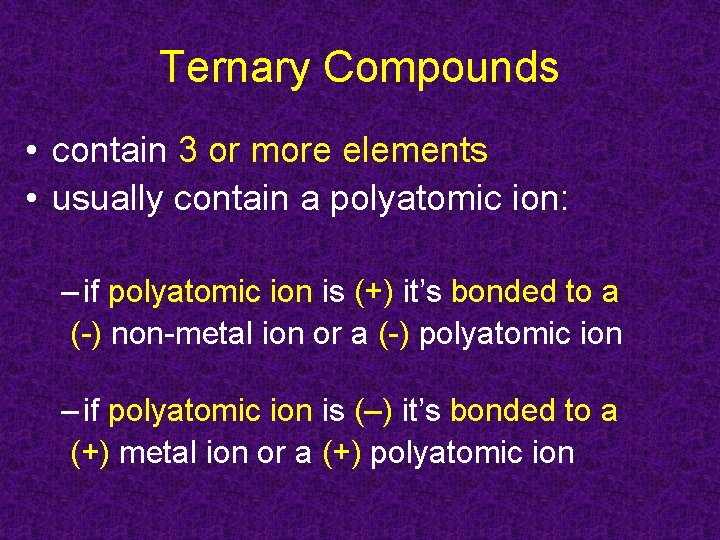Ternary Compounds • contain 3 or more elements • usually contain a polyatomic ion: – if polyatomic ion is (+) it’s bonded to a (-) non-metal ion or a (-) polyatomic ion – if polyatomic ion is (–) it’s bonded to a (+) metal ion or a (+) polyatomic ion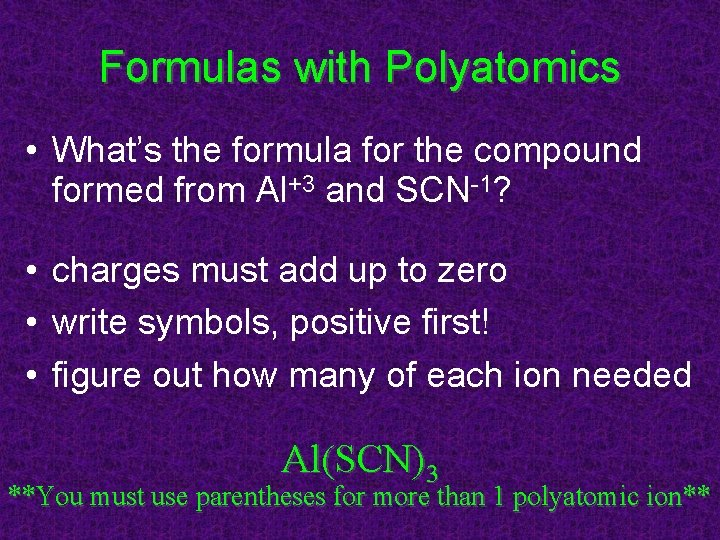Formulas with Polyatomics • What’s the formula for the compound formed from Al+3 and SCN-1? • charges must add up to zero • write symbols, positive first! • figure out how many of each ion needed Al(SCN)3 **You must use parentheses for more than 1 polyatomic ion**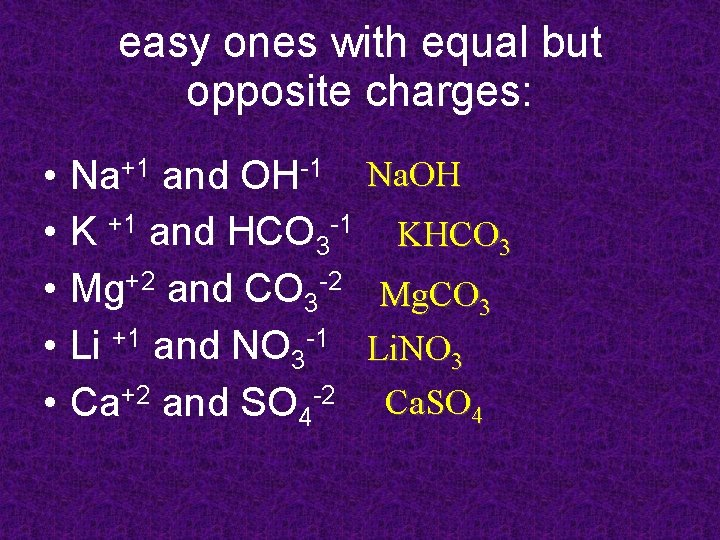easy ones with equal but opposite charges: • • • Na+1 and OH-1 K +1 and HCO 3 -1 Mg+2 and CO 3 -2 Li +1 and NO 3 -1 Ca+2 and SO 4 -2 Na. OH KHCO 3 Mg. CO 3 Li. NO 3 Ca. SO 4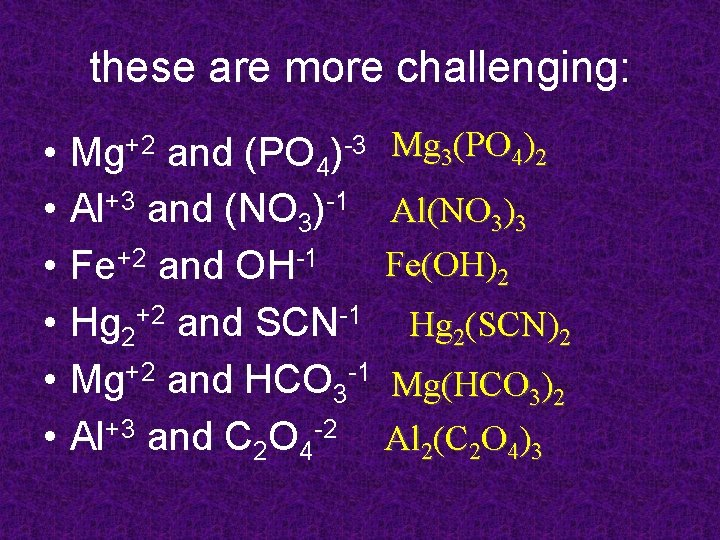these are more challenging: • • • Mg+2 and (PO 4)-3 Al+3 and (NO 3)-1 Fe+2 and OH-1 Hg 2+2 and SCN-1 Mg+2 and HCO 3 -1 Al+3 and C 2 O 4 -2 Mg 3(PO 4)2 Al(NO 3)3 Fe(OH)2 Hg 2(SCN)2 Mg(HCO 3)2 Al 2(C 2 O 4)3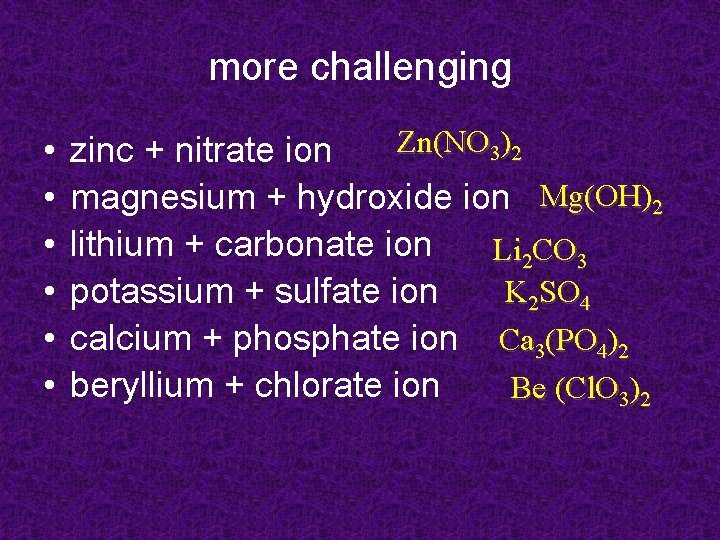more challenging • • • Zn(NO 3)2 zinc + nitrate ion magnesium + hydroxide ion Mg(OH)2 lithium + carbonate ion Li 2 CO 3 K 2 SO 4 potassium + sulfate ion calcium + phosphate ion Ca 3(PO 4)2 beryllium + chlorate ion Be (Cl. O 3)2